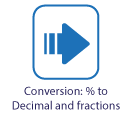# Copy of DIY Maths: Converting percents to decimals

## CONVERTING PERCENTS TO DECIMALSWriting a as a decimal is very useful for quick percentage calculations. For example 32% of 420 is calculated by 0.32 x 420. On this page you will learn how to answer questions like:

• What is 20% as a decimal?
• What is 125% as a decimal?

This slide show will show how, and why, we can write a percentage as a decimal:

This is a video showing how to convert a percent to a decimal number and back again:

## Did you know?

Changing a percentage to a decimal number is a simple as taking off the percent sign and moving the decimal point two places to the left!  For example, 32.8% = .328, and 29% = .29!

Study and practice

## Write percentage as... calculator

Use this calculator for problems like:

• What is 20% as a decimal? or
• What is 20% as a fraction?